# How to convert SOP into POS or POS into SOP ?

#### Xenon02

Joined Feb 24, 2021
324
Hello !

I've got a problem. Because I don't know how to convert SOP into POS or POS into SOP.
I've read that to convert SOP into POS I have to use double negation. But in some examples I see people are using only single negation.

For example here :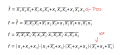But I received information from my teacher that If I want to convert SOP into POS or POS into SOP then I should use double negation or nothing.
But here he used only one negation.

I tried to change this POS :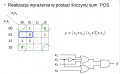Into this SOP :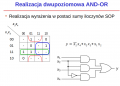It looks like that :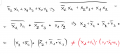But I don't know it isn't the same.
I don't know why. And I saw some sources that used double negation and those that used single negation. And I'm confused.

#### WBahn

Joined Mar 31, 2012
26,834
I'm not sure what you are talking about when you say single or double negation.

In the first example you have, notice that the SOP equation is for the inverse of f and not for f itself. So negating both sides gets you an expression for f.

But in the bottom you are starting with an SOP equation for y itself. Then you are negating it once, so if you do everything correctly, you would end up with a POS expression for the inverse of y and not for y.

#### Xenon02

Joined Feb 24, 2021
324
I'm not sure what you are talking about when you say single or double negation.
This is what I mean by double negation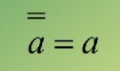Two lines at letter A Is that I use two negatings. And it is the same as the A with no negatings.

But I heard from my teached that if I want to have SOP from POS I should use two negatings or nothing, and not a single one like in the examples. But here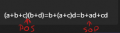he didn't use a single negatings. He said also that if I do it correctly the POS and SOP should give the same results

But in the bottom you are starting with an SOP equation for y itself. Then you are negating it once, so if you do everything correctly, you would end up with a POS expression for the inverse of y and not for y.
I gave how did I calculate the bottom one and It didn't work. I don't know where did I make a mistake.

It's more like I see from my teacher examples where he uses one negation to change POS to SOP and one time I see he doesn't use any negations. He said also that if I use only one negation I'll change the changes boolean values. So he said "if you want to convert POS into SOP or SOP into POS then use double negatings or nothing. Single negatings will change the boolean values".

#### WBahn

Joined Mar 31, 2012
26,834
It sounds like you don't really know what negation is. It makes whatever you negate the opposite of what it was. So double negation does this twice. But if you negate something twice, you end up right back where you started.

In your first example, the function at the top is f' (f with a bar over it). That means that the expression on the right gives the opposite of f. So if you negate that once, you end up with the opposite of the opposite of f, which is just f.

In your bottom example, you are starting with an expression for y. So if you negate that just once, you end up with something that yields the opposite of y. You would need to negate that again to get an expression that is equivalent to y.

#### Xenon02

Joined Feb 24, 2021
324
Or maybe this is POS for "1" output and if I want POS for "0" output ? I need to add negation ?
I don't know because my teacher once calculated POS and SOP and said I don't need negation I just need to change it like here :And on other pictures he uses negation like here:#### WBahn

Joined Mar 31, 2012
26,834
The bottom one wasn't negated

View attachment 273983

My teacher said that this is converted POS to SOP. He didn't used a single negation. Also in other examples he had to use one negation.
This is nothing more than using the distributive property and a couple others.

(a+b+c)(b+d)
(a+b+c)(b) + (a+b+c)(d) Distribute the AND over the OR
ab + bb + cb + ad + bd + cd Distribute the AND over the OR for both terms
ab + bb + cb + ad + db + cd Commutative property of AND (bd = db)
ab + b + cb + ad + db + cd Idempotence of AND (bb = b)
ab + 1b + cb + ad + db + cd Identity property of AND (b = 1b)
ab + 1b + cb + db + ad + cd Commutative property of OR (ad + db = db + ad)
(a + 1 + c + d)b + ad + cd Distributive property of AND over OR (ab + 1b + cb + db = (a + 1 + c + d)b)
(1)b + ad + cd Dominance property of OR (1+x = 1)
b + ad + cd

#### WBahn

Joined Mar 31, 2012
26,834
Or maybe this is POS for "1" output and if I want POS for "0" output ? I need to add negation ?

And on other pictures he uses negation like here:

View attachment 273989
Look at that top line. That is an equation in POS form that is for f', not for f. If you negate that equation, you end up with an equation for f. It happens to be an equation in a form for which it is straight forward to apply De Morgan's Theorem in order to get it in SOP form.

You need to look at what you have, not just blindly throw some memorized steps at it.

#### Xenon02

Joined Feb 24, 2021
324
So this one :Is a convertion from POS to SOP ? A correct convertion ?So If I want to change this SOP into POS I don't need to add negation because on the first one there is no negation. If I change this SOP to POS without negation then it is POS for f not for f' yes ? If this is POS for f not for f' then this is POS from "1" output but if this is POS for f' then this is for "0" output.

I know that from karnaugh when I take SOP and I want it to converte into POS I need to add negation. But I don't know why because here :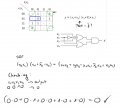So why do I have to add negation ? Here I didn't add negation and the output was correct. But in many tutorials people says that I need for POS taken from Karnaugh equation add negation to change it into SOP. You also mentioned to add negation to change SOP into POS or POS into SOP. But now it is not correct.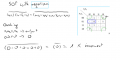Last edited:

#### WBahn

Joined Mar 31, 2012
26,834
You are still trying to follow some memorized mantra instead of understanding what is going on.

All POS and SOP are are just two possible forms that a Boolean equation can take on. That's it. They are just Boolean equations written in a particular form.

If I have the equation

Y = (A + B)(AC + B) + BC

That is just a Boolean equation describing the Truth Table for a Boolean function named Y. It happens to be in neither POS nor SOP form.

If I perform some operations on it I can get it into other many other forms -- there are actually an infinite number of equivalent forms. Some of those forms will be in POS and some will be in SOP.

If I negate both sides, I have

Y' = [(A + B)(AC + B) + BC]'

The right side is still just a Boolean equation, but does NOT describe the function named Y, but rather the inverse of it. Everywhere that Y is True, this equation will the False, and vice versa.

Karnaugh maps are simply a graphical mechanism for helping us to craft Boolean equations. In particular, they make it pretty easy to make equations in either SOP or POS form.

To make an equation in POS form, we identify rectangular regions that are all True and write a term that is the AND of the inputs that describe that region. If we make sure that we use enough regions to cover all of the True entries in the map, we can just OR all of the terms together and we have an equation for the function described by the map that is written in SOP form.

Making the equation that describes the map in POS form is less obvious, but in reality all we are doing is finding the SOP form for the inverse of the map and then applying De Morgan's theorem.

#### Xenon02

Joined Feb 24, 2021
324
Aha okey I think I get it so here :I have SOP for function named f, and I can make POS just using simple twist in equation withouth using negation. If I use negation for this SOP then I will get just the SOP inverse of function f.

By the way from the karnaugh for POS here :This equation (X2+X4)(X1+~X2+X3) is for funtion y

And for SOP here :This is inverse of y ? or is it normal y like for the POS ?

Last edited:

#### WBahn

Joined Mar 31, 2012
26,834

#### Xenon02

Joined Feb 24, 2021
324
Yes I know I mean if this POS (from the last line) can be changed into SOP using De Morgana. and I will get SOP for f not for f with upper line.

I undestand now that those f and f with upper line are not the same. What I mean now is that this last line POS can be now changed into SOP without negating because I will get SOP for function f.

Because on top there is SOP for function f with upper line so can I have SOP for function f ?

#### WBahn

Joined Mar 31, 2012
26,834
Because on top there is SOP for function f with upper line so can I have SOP for function f ?
NO!

The top line is SOP for f', but the bottom line is POS (and NOT SOP!) for f.

SOP => SUM of PRODUCTS => (product #1) + (product #2) + (product #3) + ... + (product #n)
POS => PRODUCT of SUMS => (sum #1)(sum #2)(sum #3)...(sum #n)

#### Xenon02

Joined Feb 24, 2021
324
My english is pretty bad SOP for function f is exactly what you said : SOP for f'. So I will use now f'.

I mean all the time that from the final product which is POS = (X1+X2+X3)*(X1+~X2+X3)*(~X1+X2+X3)*(~X1+X2+~X3).
Can I make this POS which is for f into SOP also for f and not for f' ?

Last edited:

#### WBahn

Joined Mar 31, 2012
26,834
My english is pretty bad SOP for function f is exactly what you said : SOP for f'. So I will use now f'.

I mean all the time that from the final product which is POS = (X1+X2+X3)*(X1+~X2+X3)*(~X1+X2+X3)*(~X1+X2+~X3).
Can I make this POS which is for f into SOP also for f and not for f' ?
Sure.

You can always just "multiply" it out and eliminate redundant terms.

Another option is to identify which maxterms are included in the POS equation and then recognize that the SOP equation consists of the minterms for the missing maxterms.

f = (X1+X2+X3)*(X1+~X2+X3)*(~X1+X2+X3)*(~X1+X2+~X3)

When a POS equation consists solely of maxterms, it is known as being in canonical form. A maxterm is an expression consisting of an OR every every variable, some of which may be complemented.

The easiest way I can think of off the top head to identify the POS maxterms is to expand each factor that is NOT a maxterm into an equivalent set of maxterms.

Your POS equation for f happens to be in canonical form, but let's "simplify" this equation a bit to a more typical starting point and then walk ourselves back to canonical form and transform from there to SOP.

For example, your last two factors could be combined to yield:

f = (X1+X2+X3)*(X1+~X2+X3)*(~X1+X2)

while the first two can be combined to yield:

f = (X1+X3)*(~X1+X2)

So let's convert this to canonical form.

Neither of these factors is a maxterm since variables are missing. X2 is missing from the first and X3 is missing from the second. To expand these back, you just double number of each product for each missing variable and put the complemented version in one and the uncomplemented in the other. Doing that explicitly, this looks like:

f = (X1+X3)*(~X1+X2)
f = [(X1+X3)*(X2+~X2)]*[(~X1+X2)*(X3+~X3)]
f = [(X1+X3)*(X2+~X2)]*[(~X1+X2)*(X3+~X3)]
f = [(X1+~X2+X3)*(X1+X2+X3)]*[(~X1+X2+X3)*(~X1+X2+~X3)]
f = (X1+~X2+X3)*(X1+X2+X3)*(~X1+X2+X3)*(~X1+X2+~X3)

In an equation with three variables, there are 2^3 = 8 possible maxterms. We have four of them, so four are missing. Identify the missing ones:

(X1+~X2+~X3), (X1+X2+~X3), (~X1+~X2+X3), (~X1+~X2+~X3)

A function consisting of these maxterms is actually f'

f' = (X1+~X2+~X3)*(X1+X2+~X3)*(~X1+~X2+X3)*(~X1+~X2+~X3)

Now you can apply the prior process using De Morgan's Theorem to invert this into SOP form, which can be done by inspection:

f = (~X1*X2*X3) + (~X1*~X2*X3) + (X1*X2*~X3) + (X1*X2*X3)

•Xenon02

#### Xenon02

Joined Feb 24, 2021
324
Sure.

You can always just "multiply" it out and eliminate redundant terms.

Another option is to identify which maxterms are included in the POS equation and then recognize that the SOP equation consists of the minterms for the missing maxterms.

f = (X1+X2+X3)*(X1+~X2+X3)*(~X1+X2+X3)*(~X1+X2+~X3)

When a POS equation consists solely of maxterms, it is known as being in canonical form. A maxterm is an expression consisting of an OR every every variable, some of which may be complemented.

The easiest way I can think of off the top head to identify the POS maxterms is to expand each factor that is NOT a maxterm into an equivalent set of maxterms.

Your POS equation for f happens to be in canonical form, but let's "simplify" this equation a bit to a more typical starting point and then walk ourselves back to canonical form and transform from there to SOP.

For example, your last two factors could be combined to yield:

f = (X1+X2+X3)*(X1+~X2+X3)*(~X1+X2)

while the first two can be combined to yield:

f = (X1+X3)*(~X1+X2)

So let's convert this to canonical form.

Neither of these factors is a maxterm since variables are missing. X2 is missing from the first and X3 is missing from the second. To expand these back, you just double number of each product for each missing variable and put the complemented version in one and the uncomplemented in the other. Doing that explicitly, this looks like:

f = (X1+X3)*(~X1+X2)
f = [(X1+X3)*(X2+~X2)]*[(~X1+X2)*(X3+~X3)]
f = [(X1+X3)*(X2+~X2)]*[(~X1+X2)*(X3+~X3)]
f = [(X1+~X2+X3)*(X1+X2+X3)]*[(~X1+X2+X3)*(~X1+X2+~X3)]
f = (X1+~X2+X3)*(X1+X2+X3)*(~X1+X2+X3)*(~X1+X2+~X3)

In an equation with three variables, there are 2^3 = 8 possible maxterms. We have four of them, so four are missing. Identify the missing ones:

(X1+~X2+~X3), (X1+X2+~X3), (~X1+~X2+X3), (~X1+~X2+~X3)

A function consisting of these maxterms is actually f'

f' = (X1+~X2+~X3)*(X1+X2+~X3)*(~X1+~X2+X3)*(~X1+~X2+~X3)

Now you can apply the prior process using De Morgan's Theorem to invert this into SOP form, which can be done by inspection:

f = (~X1*X2*X3) + (~X1*~X2*X3) + (X1*X2*~X3) + (X1*X2*X3)
Cool so I can have SOP for f function (not for f') out of POS. And I thought exactly about multiplying, but your method is interesting. I'll try to learn it laterAlso the SOP here :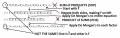My teacher said he took it from karnaugh mapping for "0" output. And I thought why is this SOP inverted

So is this karnaugh map which shows SOP equation :Is it also inverted ? I mean is it f' ?

Because POS here is f as I know :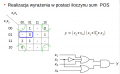#### Xenon02

Joined Feb 24, 2021
324
Last edited:

#### WBahn

Joined Mar 31, 2012
26,834
This POS is in f function or is it in f' reversed to that SOP ? ( mean those karnaugh mappings I send above).

Or maybe better question how to read from Karnaugh map POS for reversed function.
The K-maps you have in Post #17 don't have an f or f' -- the function name there is y.

Both expressions are for y (which is why both start with "y = ....").

First off, do you understand how to get the SOP equation from the top K-map?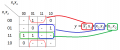Make sure that you understand how each of those three terms were obtained from the groupings in the K-map.

Once you are comfortable with that, look at the groupings of the zeros. These are where the function y is False or, in other words, where y' is True. Use the procedure as above to create an SOP equation for y'.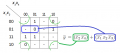Now negate both sides and then apply De Morgan's Theorem and then apply it again to each factor that results from the first application: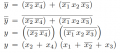Do you now see where the POS equation comes from and how you can write it down by inspection by grouping the zeros in the K-map and then, for each group, creating a factor that uses the opposite of what you would use for each variable if you were making the SOP equation.

•Xenon02